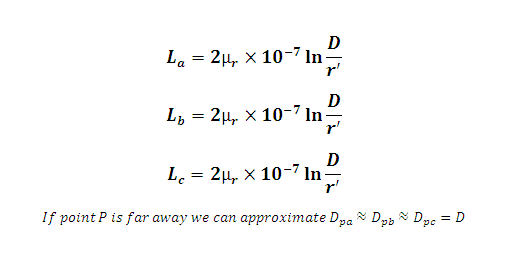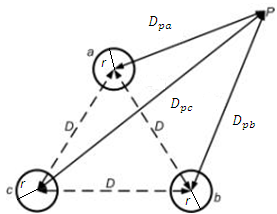# Inductance of Symmetrical Three Phase Transmission Line Calculator

This Calctown Calculator calculates the inducatnce of symmetrically spaced three phase transmission line.

Here µ0 = 4p * 10-7. Please enter the RELATIVE permeability accordingly.

m
m
unit

#### Result

Hwhere

La, Lb, Lc are individual phase inductance

Dpa, Dpb, Dpc are individual line distances from point P assumed to be equal for all the lines.

D = Distance between the lines